# 【综述】神经网络中不同类型的卷积层

### 1. Convolution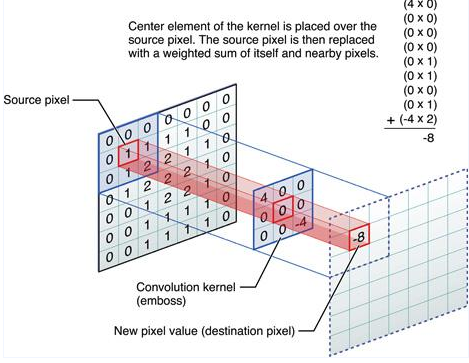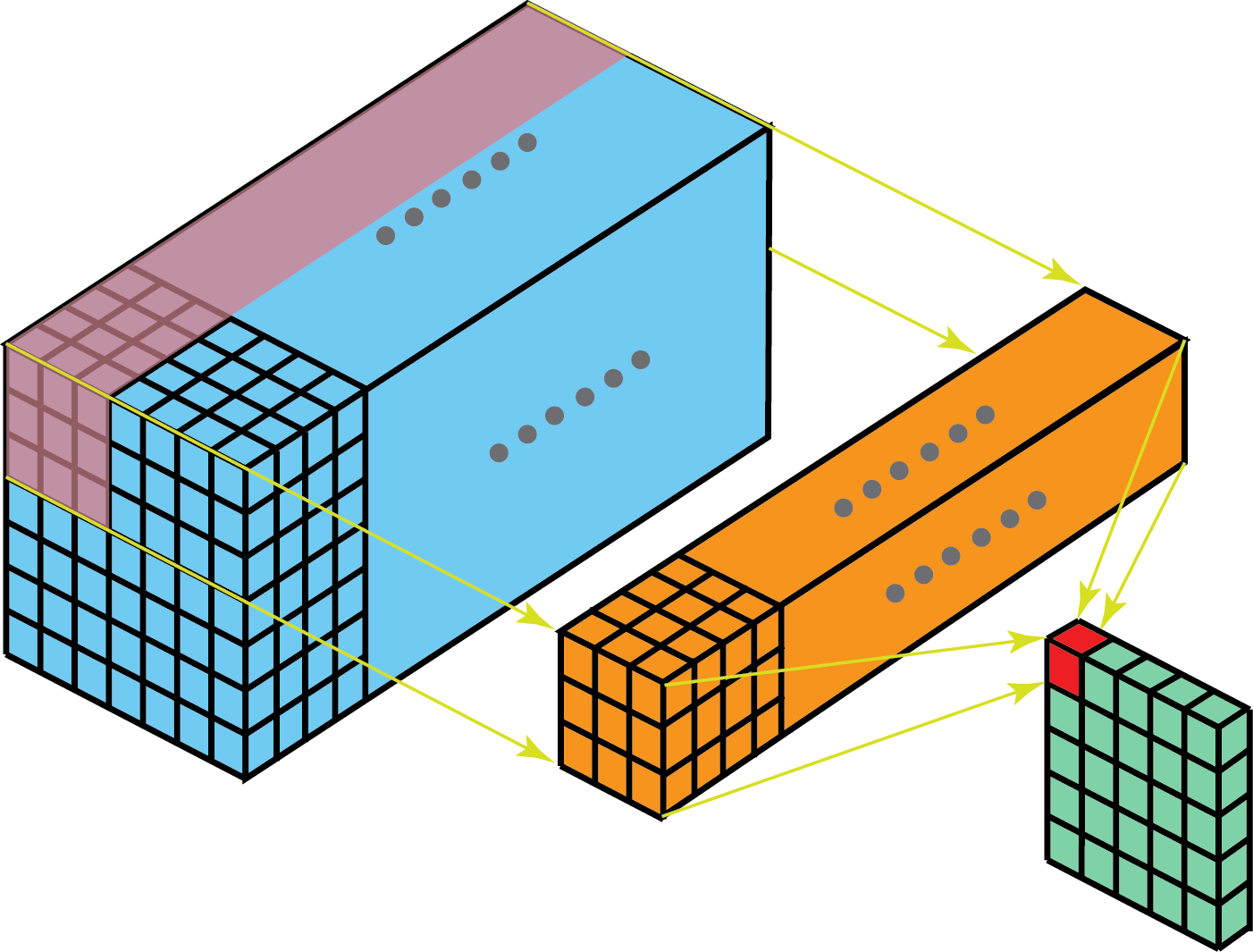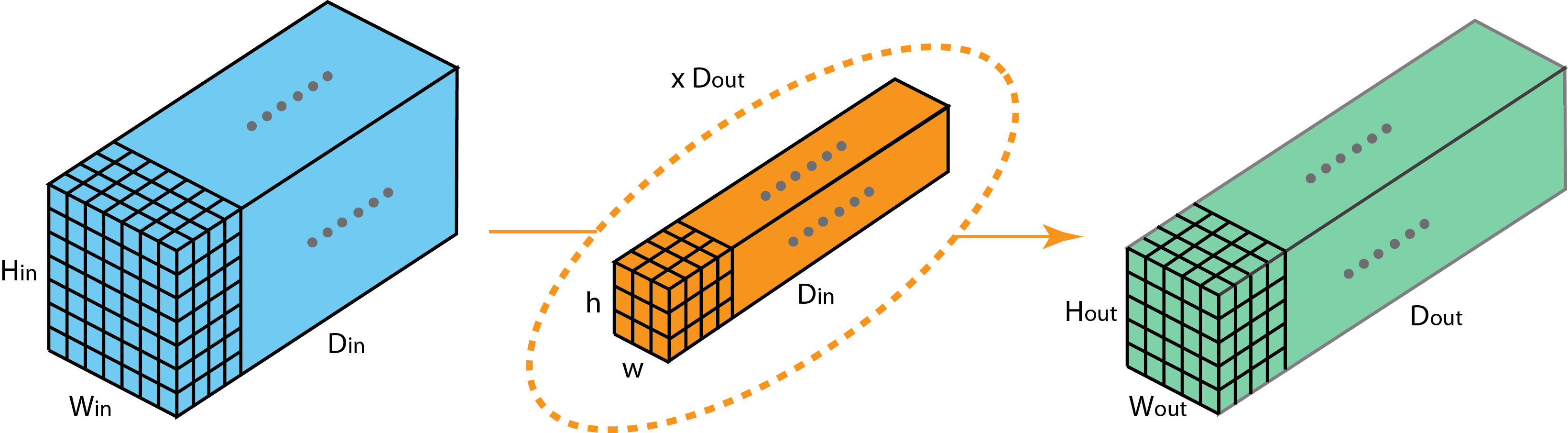### 2. 1x1/Pointwise Convolutions

• 用于降维或者升维，可以灵活控制特征图filter个数
• 减少参数量，特征图filter少了，参数量也会减少。
• 实现跨通道的交互和信息整合。
• 在卷积之后增加了非线性特征（添加激活函数）。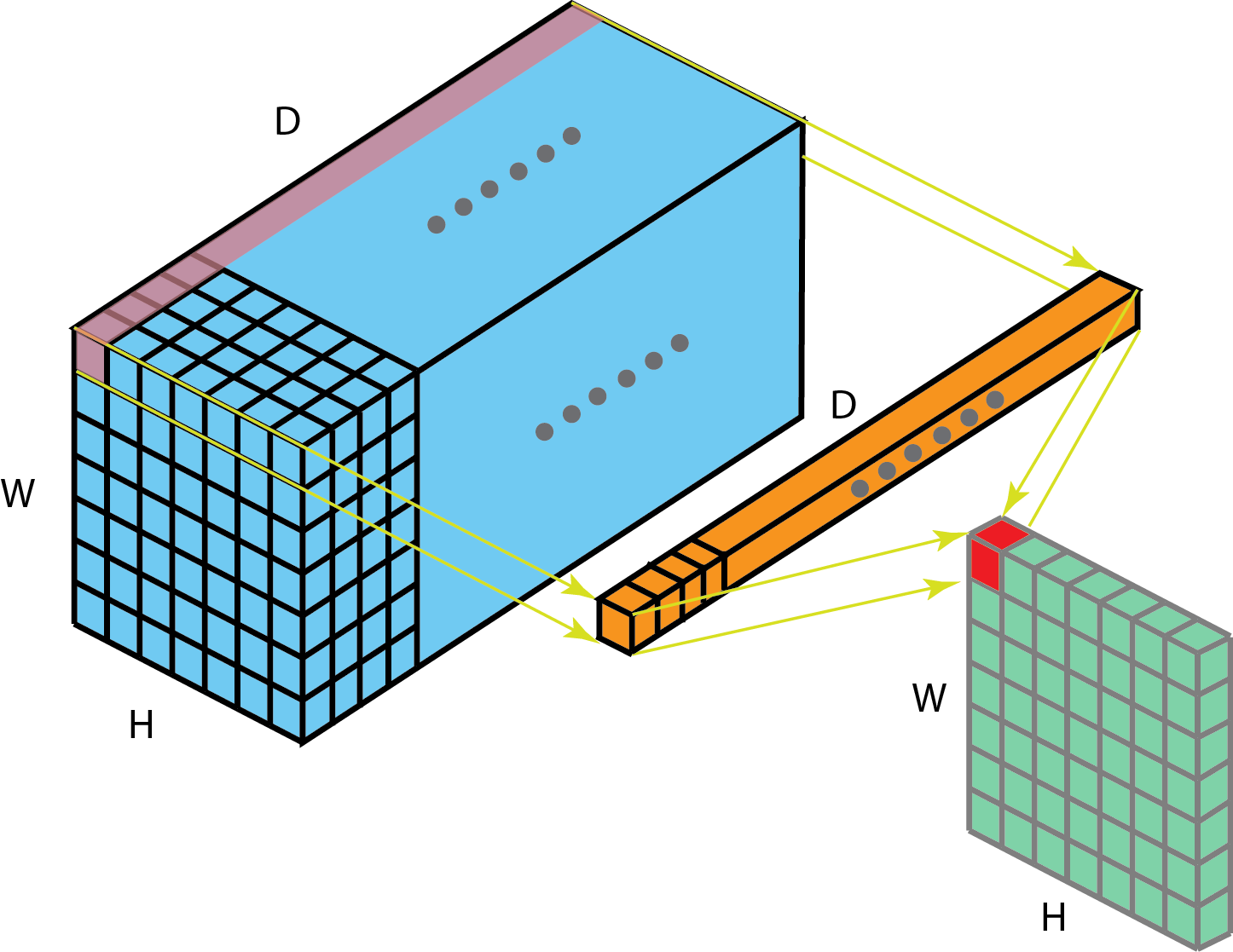### 3. Spatial and Cross-Channel Convolutions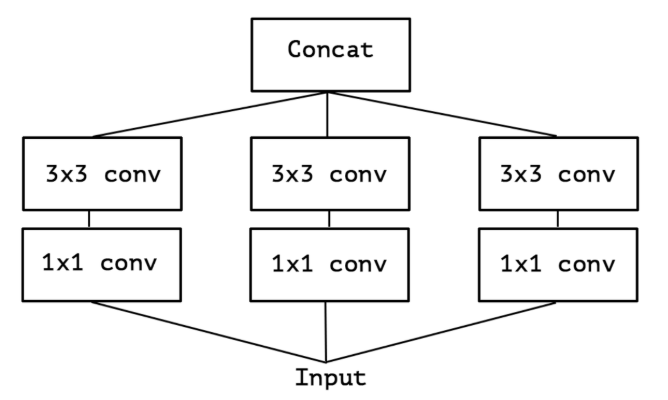pytorch实现（上图只是简化图，和代码并不一一对应）

class InceptionA(nn.Module):
def __init__(self, in_channels, pool_features, conv_block=None):
super(InceptionA, self).__init__()
if conv_block is None:
conv_block = BasicConv2d
self.branch1x1 = conv_block(in_channels, 64, kernel_size=1)

self.branch5x5_1 = conv_block(in_channels, 48, kernel_size=1)
self.branch5x5_2 = conv_block(48, 64, kernel_size=5, padding=2)

self.branch3x3dbl_1 = conv_block(in_channels, 64, kernel_size=1)
self.branch3x3dbl_2 = conv_block(64, 96, kernel_size=3, padding=1)
self.branch3x3dbl_3 = conv_block(96, 96, kernel_size=3, padding=1)

self.branch_pool = conv_block(in_channels, pool_features, kernel_size=1)

def _forward(self, x):
branch1x1 = self.branch1x1(x)

branch5x5 = self.branch5x5_1(x)
branch5x5 = self.branch5x5_2(branch5x5)

branch3x3dbl = self.branch3x3dbl_1(x)
branch3x3dbl = self.branch3x3dbl_2(branch3x3dbl)
branch3x3dbl = self.branch3x3dbl_3(branch3x3dbl)

branch_pool = F.avg_pool2d(x, kernel_size=3, stride=1, padding=1)
branch_pool = self.branch_pool(branch_pool)

outputs = [branch1x1, branch5x5, branch3x3dbl, branch_pool]
return outputs

def forward(self, x):
outputs = self._forward(x)


### 4. Grouped Convolutions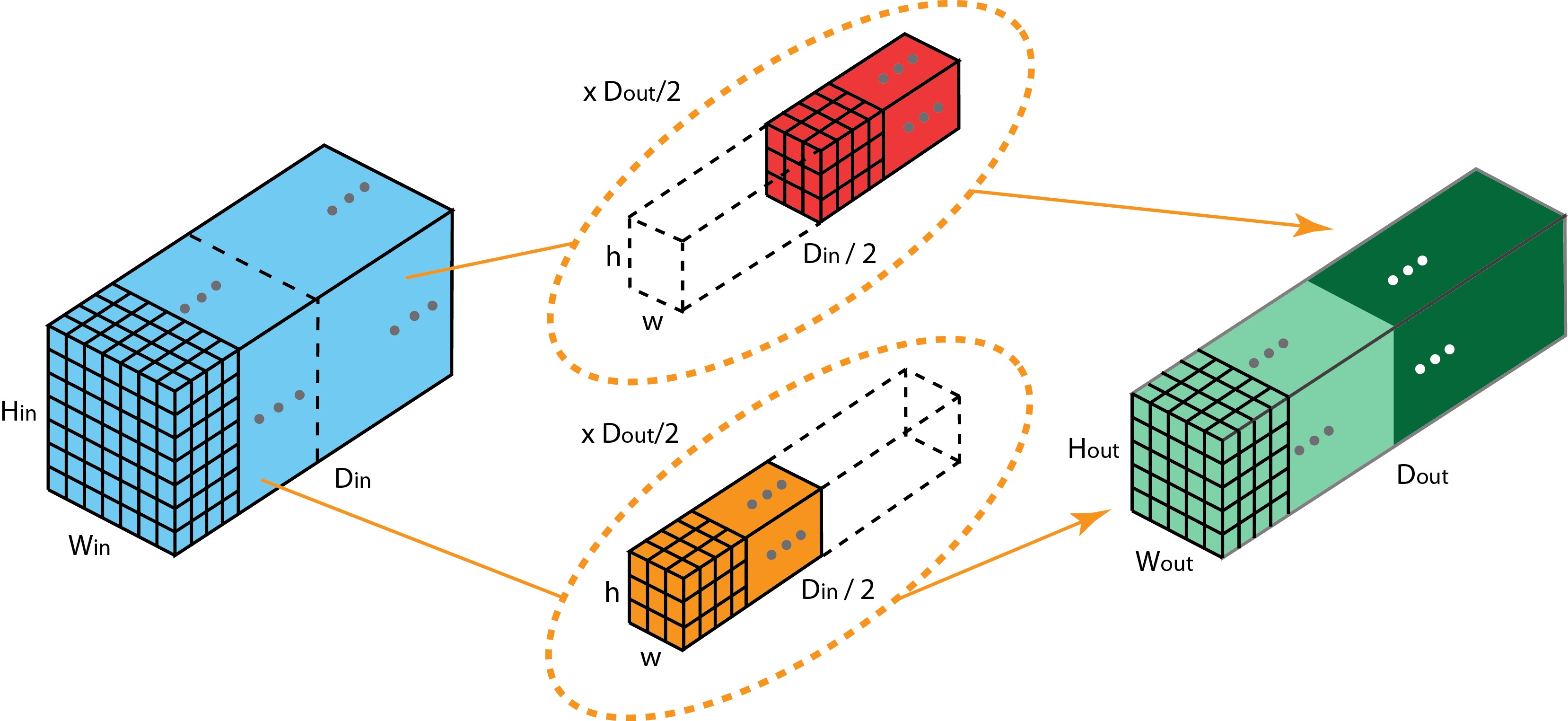ResNeXt是ResNet和Inception的结合，其每个分支都采用的相同的拓扑结构。ResNeXt本质是使用组卷积（Grouped Convolutions），通过基数( cardinality )来控制组的数量。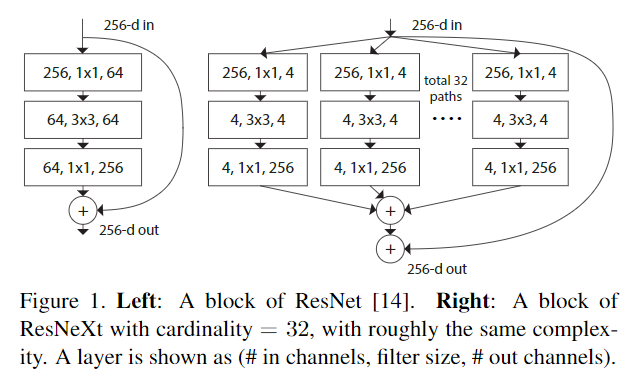• 训练效率高。由于卷积被分为几个不同的组，每个组的计算就可以分配给不同的GPU核心来进行计算。这种结构的设计更符合GPU并行计算的要求，这也能解释为何ResNeXt在GPU上效率要高于Inception模块。

• 模型效率高。模型参数随着组数或者基数的增加而减少。

• 效果好。分组卷积可能能够比普通卷积组成的模型效果更优，这是因为滤波器之间的关系是稀疏的，而划分组以后对模型可以起到一定正则化的作用。从COCO数据集榜单就可以看出来，有很多是ResNeXt101作为backbone的模型在排行榜非常靠前的位置。

组卷积为何效果更好的详细解释可以看这篇博客：https://blog.yani.io/filter-group-tutorial/，其中有比较详细的解释。

### 5. Separable Convolutions

• 空间也就是指：[height, width]这两维度组成的。
• 深度也就是指：channel这一维度。

#### 5.1 Spatially Separable Convolutions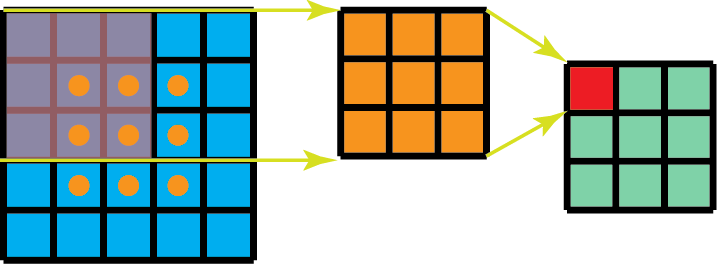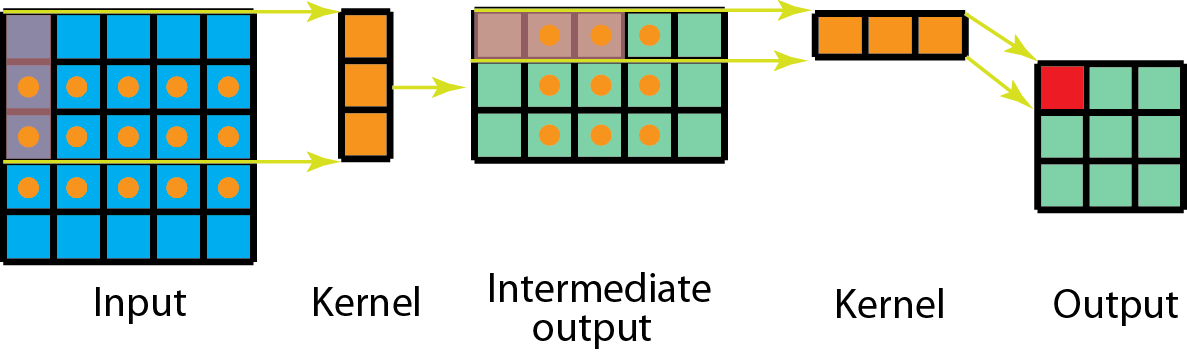$(n-2)\times(n-2)\times m\times m$

$n\times(n-2)\times m+(n-2)\times (n-2)\times m = 2(n-1)(n-2)m$

$\frac{Spatially Separable Convolution}{StandardConvolution}=\frac{2}{m}+\frac{2}{m(n-2)}$

#### 5.2 Depthwise Separable Convolutions

• Depthwise Convolution: 独立地施加在每个通道的空间卷积
• Pointwise Convolution: 1x1 convolution,通过深度卷积将通道输出投影到一个新的通道空间。

class DWConv(nn.Module):
def __init__(self, in_plane, out_plane):
super(DWConv, self).__init__()
self.depth_conv = nn.Conv2d(in_channels=in_plane,
out_channels=in_plane,
kernel_size=3,
stride=1,
groups=in_plane)
self.point_conv = nn.Conv2d(in_channels=in_plane,
out_channels=out_plane,
kernel_size=1,
stride=1,
groups=1)
def forward(self, x):
x = self.depth_conv(x)
x = self.point_conv(x)
return x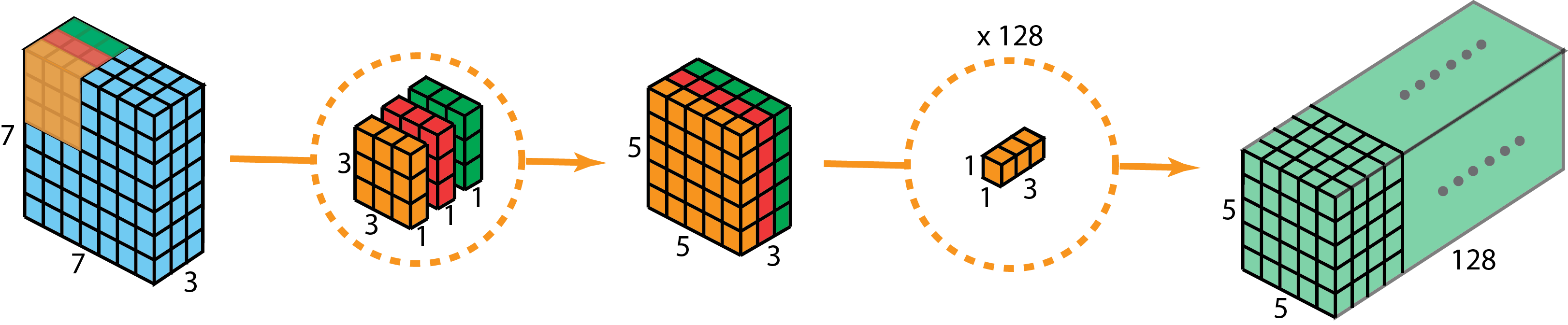Inception模块和可分离卷积的区别：

• 可分离卷积是先用Depthwise Convolution, 然后再使用1x1卷积；Inception中是先使用1x1 Convolution，然后再使用Depthwise Convolution。
• 深度可分离卷积实现的时候没有增加非线性特征（也就是使用激活函数）。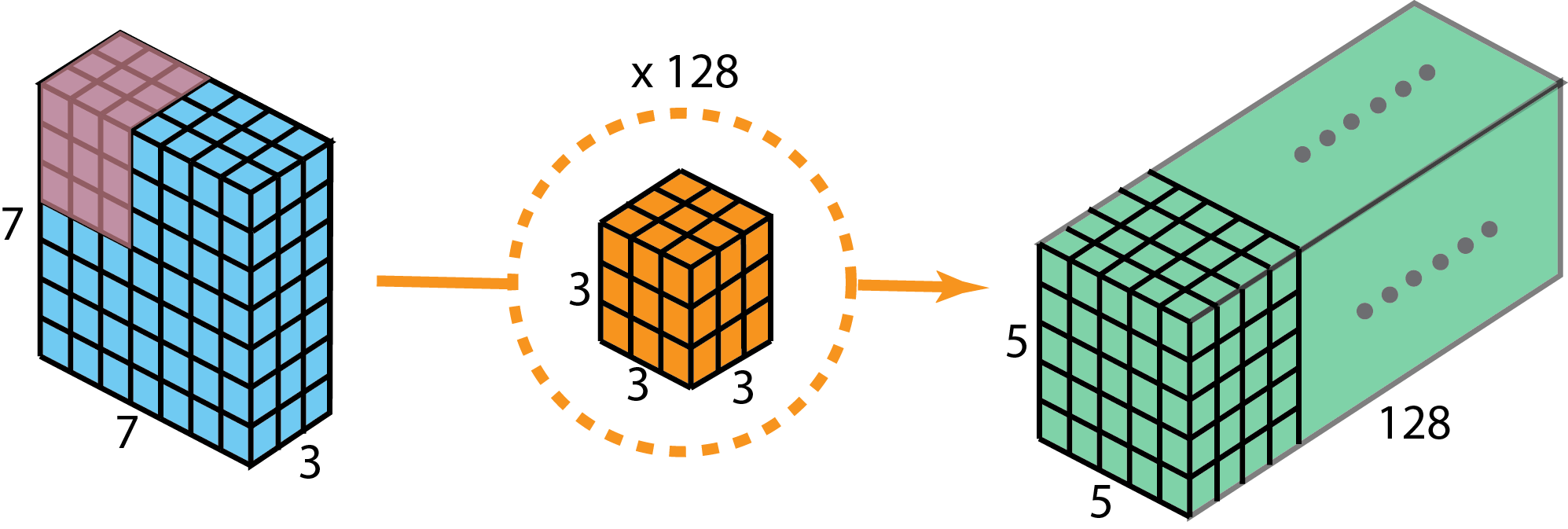$128\times(3\times3\times3)\times(5\times5)=86400$

$3\times3\times3\times1\times5\times5=675$

$128\times1\times1\times3\times5\times5=9600$

$N_c\times h\times h\times C\times (H-h+1)\times (W-h+1)$

$DepthConv = C\times h\times h\times 1\times (H-h+1)\times (W-h+1)$

$PointConv = N_c\times 1\times 1\times C\times (H-h+1)\times (W-h+1)$

$DepthwiseSeparableConv=(h\times h+N_c)\times C\times (H-h+1)\times (W-h+1)$

$\frac{DepthwiseSeparableConvolution}{StandardConvolution}=\frac{1}{N_c}+\frac{1}{h^2}$

### 6. Flattened Convolutions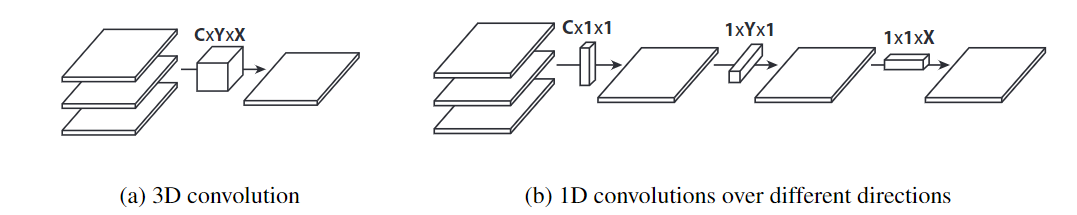### 7. Shuffled Grouped Convolutions

Channel Shuffle操作主要是为了消除原来Grouped Convolution中存在的副作用，也就是输出feature map的通道仅仅来自输入通道的一小部分，因此每个滤波器组仅限于学习一些特定的特性，如下图（a）所示。

Grouped Convolution的这个属性会阻碍信息在通道组之间的信息流动并削弱了模型的表达。通过使用Channel Shuffle可以促进通道间信息的融合从而解决以上问题。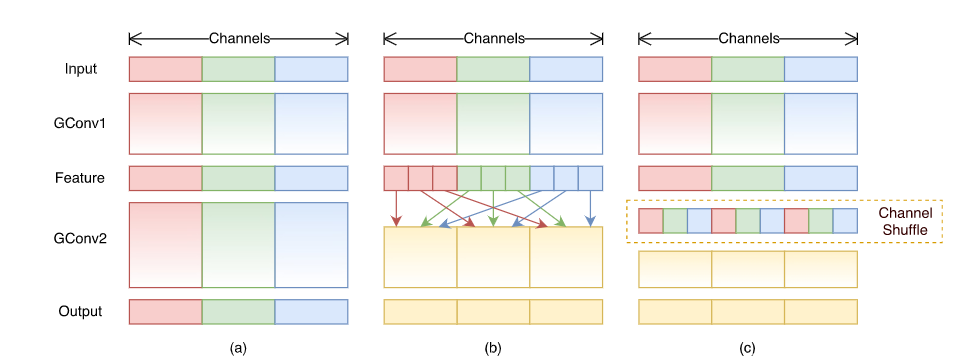ShuffleNet还用到了pointwise grouped convolution, 作者认为1x1卷积成本也非常高，所以也对1x1卷积使用组卷积，具体模块化的设计如下图(b)和(c)所示。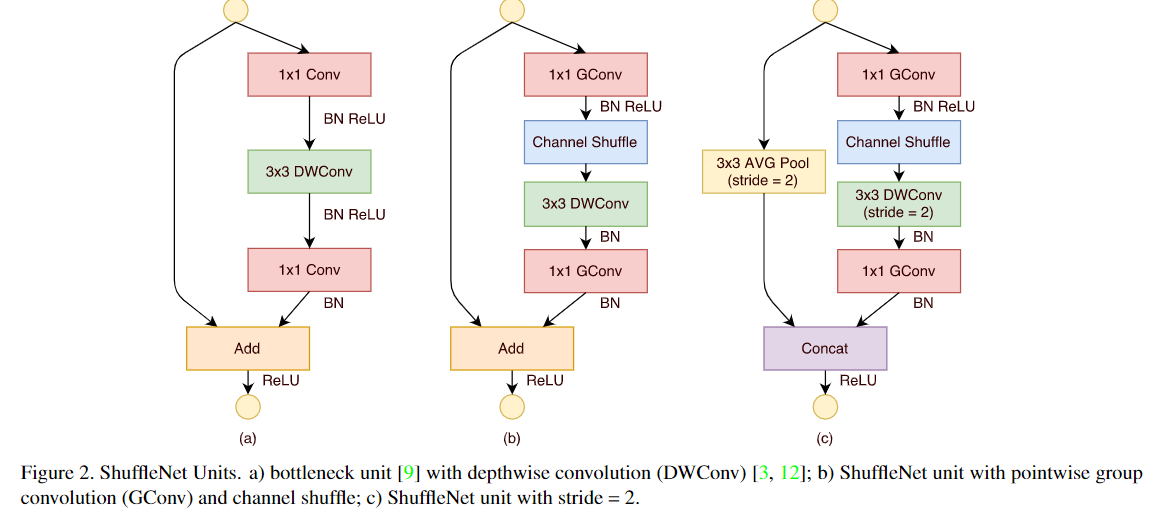• shuffled grouped convolution = grouped convolution + Channel Shuffle
• pointwise grouped convolution = 1x1 convolution + grouped convolution
• depthwise separable convolution

### 8. Dilated Convolution(Atrous Convolution)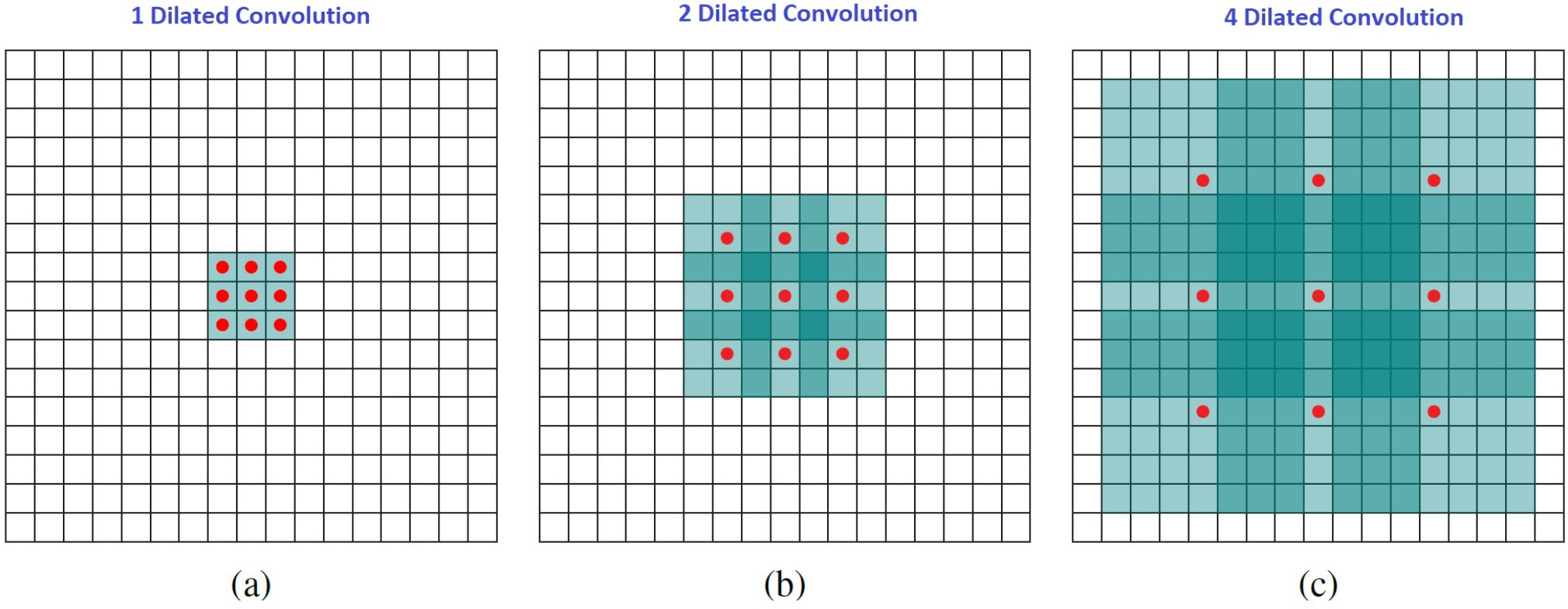• Gridding Effect: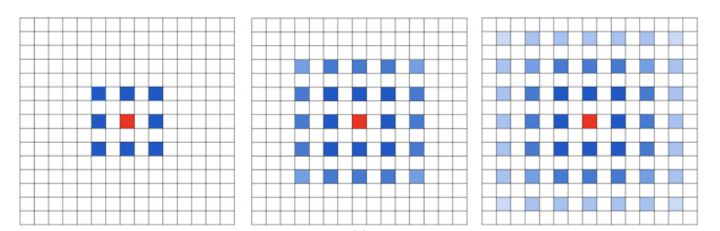• 上下文信息多少

dilated convolution可以获取长距离信息，使用dilated convolution对大物体的效果会有一定效果，但是对小物体来说并不友好，小物体所需要的感受野并不需要太大。所以如何同时处理好不同大小物体之间的关系是使用空洞卷积的关键。

### 9. Deformable Convolution

DCN提出的动机是为何特征提取形状一定要是正方形或者矩形，为何不能更加自适应的分布到目标上（如下图右侧所示），进而提出了一种形状可学习的卷积。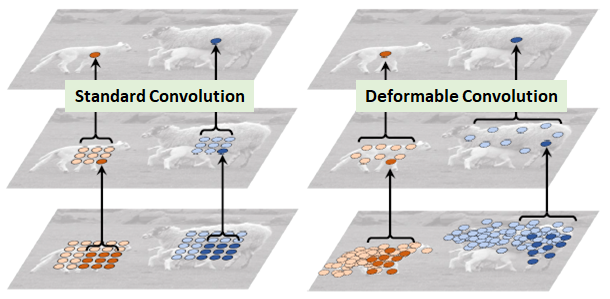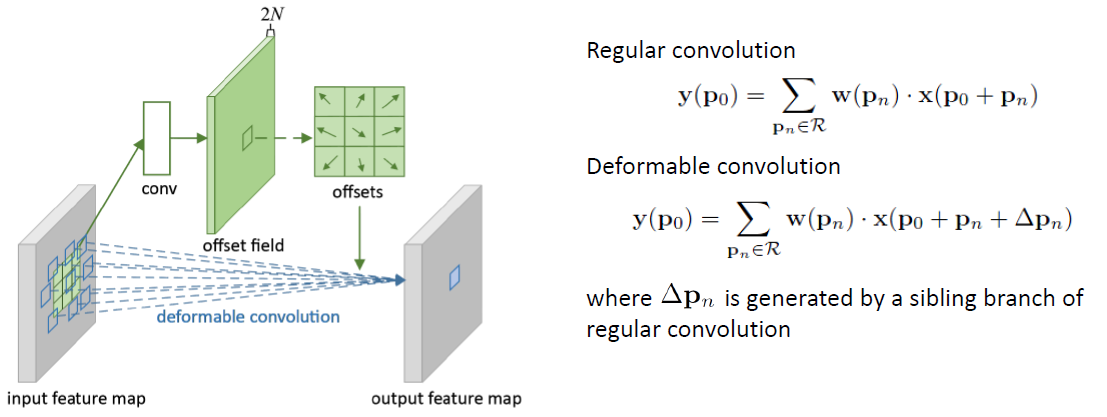### 10. Attention

#### 10.1 Squeeze and Excitation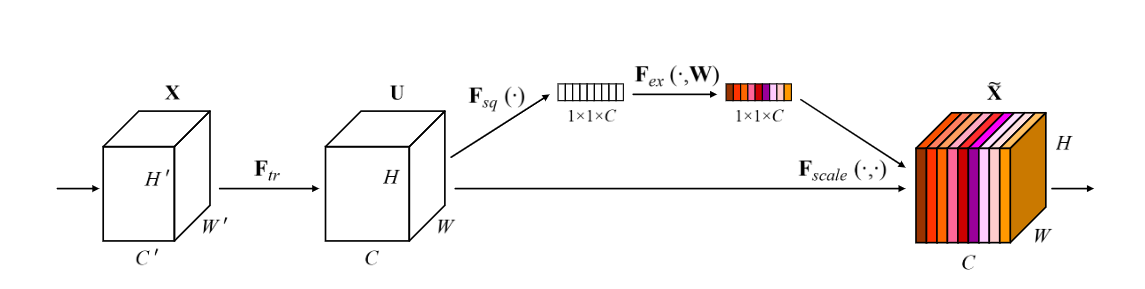SENet的核心想法是，每个通道的重要性不是一样的。基于这个想法，SENet添加了一个模块，如上图靠上的分支，这个模块作用是给每个通道打分，最终将打分的结果与卷积得到的feature map相乘，完成特征通道的权重重分配。SENet是通道注意力机制的最经典的实现。

pytorch实现：

class SELayer(nn.Module):
def __init__(self, channel, reduction=16):
super(SELayer, self).__init__()
self.fc = nn.Sequential(
nn.Linear(channel, channel // reduction, bias=False),
nn.ReLU(inplace=True),
nn.Linear(channel // reduction, channel, bias=False),
nn.Sigmoid()
)

def forward(self, x):
b, c, _, _ = x.size()
y = self.avg_pool(x).view(b, c)
y = self.fc(y).view(b, c, 1, 1)
return x * y.expand_as(x)


#### 10.2 Convolutional Block Attention Module

CBAM模块算是比较早的一批将通道注意力机制和空间注意力机制结合起来的模型，通过添加该模块，能在一定程度上优化feature。

CBAM分为Channel Attention Module 和 Spatial Attention Module: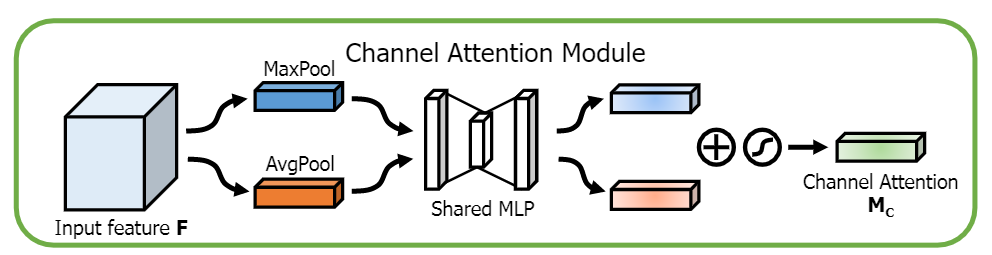pytorch实现通道注意力机制：

class ChannelAttention(nn.Module):
def __init__(self, in_planes, rotio=16):
super(ChannelAttention, self).__init__()

self.sharedMLP = nn.Sequential(
nn.Conv2d(in_planes, in_planes // ratio, 1, bias=False), nn.ReLU(),
nn.Conv2d(in_planes // rotio, in_planes, 1, bias=False))
self.sigmoid = nn.Sigmoid()

def forward(self, x):
avgout = self.sharedMLP(self.avg_pool(x))
maxout = self.sharedMLP(self.max_pool(x))
return self.sigmoid(avgout + maxout)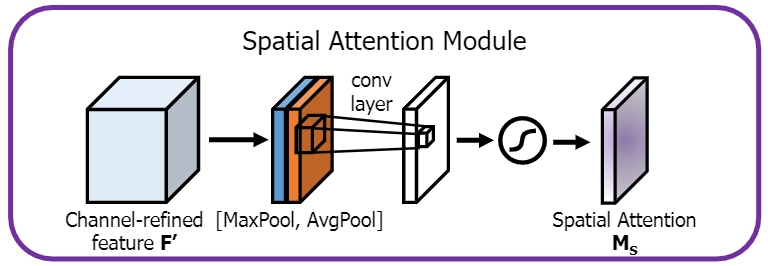pytorch实现空间注意力机制：

class SpatialAttention(nn.Module):
def __init__(self, kernel_size=7):
super(SpatialAttention, self).__init__()
assert kernel_size in (3,7), "kernel size must be 3 or 7"
padding = 3 if kernel_size == 7 else 1

self.sigmoid = nn.Sigmoid()

def forward(self, x):
avgout = torch.mean(x, dim=1, keepdim=True)
maxout, _ = torch.max(x, dim=1, keepdim=True)
x = torch.cat([avgout, maxout], dim=1)
x = self.conv(x)
return self.sigmoid(x)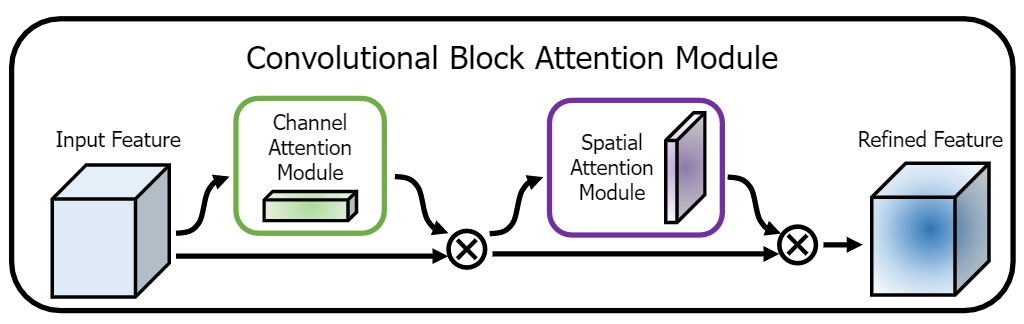### 11. Summary

• 通道和空间

• Convolution
• 1x1 Convolution
• Spatial and Cross-Channel Convolutions
• 通道相关性（channel）

• Depthwise Separable Convolutions
• Shuffled Grouped Convolutions
• Squeeze and Excitation Network
• Channel Attention Module in CBAM
• 空间相关性（HxW）

• Spatially Separable Convolutions
• Flattened Convolutions
• Dilated Convolutions
• Deformable Convolution
• Spatial Attention Module in CBAM

• 单一尺寸卷积核用多个尺寸卷积核代替（参考Inception系列）

• 使用可变形卷积替代固定尺寸卷积（参考DCN）

• 大量加入1x1卷积或者pointwise grouped convolution来降低计算量（参考NIN、ShuffleNet）

• 通道加权处理（参考SENet）

• 用深度可分离卷积替换普通卷积（参考MobileNet）

• 使用分组卷积（参考ResNeXt）

• 分组卷积+channel shuffle（参考shuffleNet）

• 使用Residual连接（参考ResNet）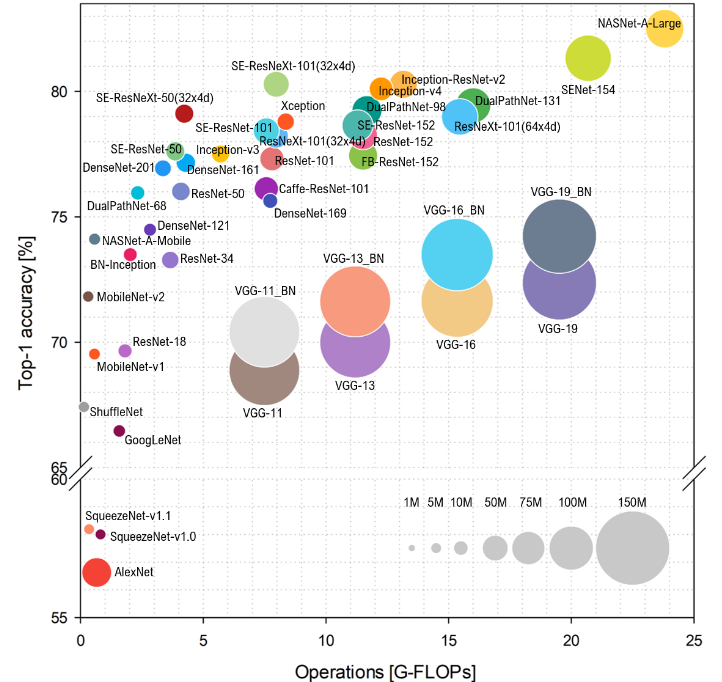## Reference

https://ikhlestov.github.io/pages/machine-learning/convolutional-layers/

https://zhuanlan.zhihu.com/p/37910136

https://ikhlestov.github.io/pages/machine-learning/convolutions-types/

https://arxiv.org/abs/1610.02357

https://arxiv.org/abs/1704.04861

https://arxiv.org/pdf/1611.05431.pdf

https://arxiv.org/abs/1707.01083

https://towardsdatascience.com/a-comprehensive-introduction-to-different-types-of-convolutions-in-deep-learning-669281e58215

http://fourier.eng.hmc.edu/e161/lectures/convolution/index.html

https://arxiv.org/pdf/1412.7062

https://arxiv.org/abs/1511.07122

https://arxiv.org/abs/1412.5474

https://www.zhihu.com/question/54149221

https://zhuanlan.zhihu.com/p/28749411

https://arxiv.org/pdf/1810.00736.pdf

posted @ 2020-03-14 19:51  pprp  阅读(1052)  评论(0编辑  收藏  举报